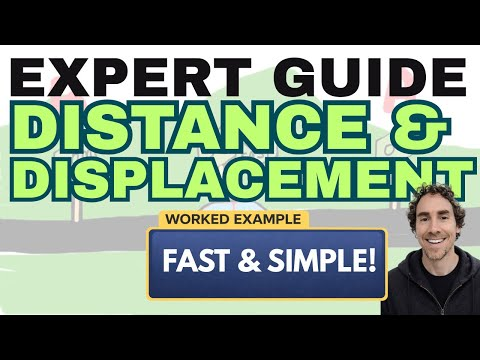### Key Difference

The main difference between distance and displacement is that the distance is the actual physical length between two points while displacement is the length of the shortest route between these two points.

### Distance vs. Displacement

Distance and displacement are two terms in Physics which are used to indicate the length between two locations, points or objects. Distance is the numerical measurement of actual length between two objects. It is a scalar quantity and is always positive. While displacement is the shortest path between two objects. It is a vector quantity and may be positive negative or zero. The SI unit of both distance and displacement is a meter (m).

### Comparison Chart

 Distance Displacement The actual length of the path between two points is known as the distance. The shortest path between two points is known as displacement. Quantity It is a scalar quantity. It is a vector quantity. Information about route It provides complete information about the route being followed. It does not provide any information about the route. Value Its value is always positive. Its value may be positive, negative or zero. Path It does not have a specific path. It has a unique path. Time Distance cannot decrease with time Displacement can decrease with time. Denoted by S d Formula S = Speed × Time d = Velocity × Time Indication It can never be indicated by the arrow. It can be indicated by the arrow. Use It can be used to calculate speed. It can be used to calculate velocity.

### What is Distance?

Distance is defined as the numerical quantity that determines the actual length between two points. Total distance can be calculated by adding all the intervals. It is only concerned with size or magnitude and ignores the direction of the path. So, it is a scalar quantity. Distance is always positive and provides complete information about the path. It always increases with time. It cannot be indicated by drawing an arrow. Distance can be calculated by multiplying the speed of the moving object with time. It is denoted by “S”.

#### Formula

Distance = Speed × Time or S = v × t

#### Example

If a person moves from a point A 5m towards the right and then 4m towards the left to reach a point B then total distance can be obtained by adding both intervals, i.e. S= 5+4 =9 So, the total distance covered by the person is 9 meters.

### What is Displacement?

Displacement is defined as the length of the shortest path between two points. It is actually changing in position and mostly in a straight line. Displacement is concerned with both the magnitude and direction of the path. So, it is a vector quantity. It does not provide any information about the route and its value may be positive, negative or even zero. It is changing in a position that matters not the route. So, if the change in position is zero than displacement will be zero. A change to the left is indicated by negative value e.g. -2m while a change to the right will be indicated by a positive value e.g. 2m. Displacement can be indicated easily by simply drawing an arrow. Displacement can be counted by multiplying velocity and time. It is denoted by “d”

#### Formula

Displacement = velocity × time or d = v × t

#### Example

If a person travels 5m towards the north and then 5m towards the south than the displacement will be zero because same distance but in opposite directions will cancel out each other.

### Key Differences

1. Distance is the length of the actual path between two points while the shortest path between these two points is known as displacement.
2. Arrow cannot be used to indicate distance while displacement can be indicated by simply drawing an arrow.
3. Distance is a scalar quantity whereas displacement is a vector quantity.
4. Distance is always positive while displacement may be positive, negative or even zero.
5. “S” is used to denote distance but “d” is used to denote displacement.
6. Displacement can be measured by multiplying speed and time whereas displacement can find out by multiplying velocity and time.
7. Complete information of the route is given by distance while displacement does not provide any information about the route.
8. Speed can find out from the distance while displacement is used to find out velocity.

### Comparison Video### Conclusion

From the above discussion, it is concluded that distance is a scalar quantity to measure exact space between two points while displacement is a vector quantity to measure the shortest path between these two points.